# Isometric operator

(diff) ← Older revision | Latest revision (diff) | Newer revision → (diff)

A mappingof a metric spaceinto a metric space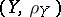such that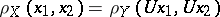for all. Ifandare real normed linear spaces,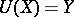and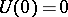, thenis a linear operator.

An isometric operatormapsone-to-one onto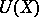, so that the inverse operator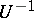exists, and this is also an isometric operator. The conjugate of a linear isometric operator from some normed linear space into another is also isometric. A linear isometric operator mappingonto the whole ofis said to be a unitary operator. The condition for a linear operatoracting on a Hilbert spaceto be unitary is the equation. The spectrum of a unitary operator (cf. Spectrum of an operator) lies on the unit circle, andhas a representation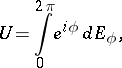whereis the corresponding resolution of the identity. An isometric operator defined on a subspace of a Hilbert space and taking values in that space can be extended to a unitary operator if the orthogonal complement of its domain of definition and its range have the same dimension.

With every symmetric operatorwith domain of definition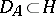is associated the isometric operator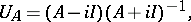called the Cayley transform of. Ifis self-adjoint, then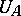is unitary.

Two operatorsandwith the same domain of definitionare said to be metrically equal if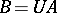, whereis an isometric operator, that is, iffor all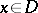. Such operators have a number of properties in common. For every bounded linear operatoracting on a Hilbert space there exists one and only one positive operator metrically equal to it, namely that defined by the equality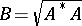.

How to Cite This Entry:
Isometric operator. Encyclopedia of Mathematics. URL: http://encyclopediaofmath.org/index.php?title=Isometric_operator&oldid=16897
This article was adapted from an original article by V.I. Sobolev (originator), which appeared in Encyclopedia of Mathematics - ISBN 1402006098. See original article